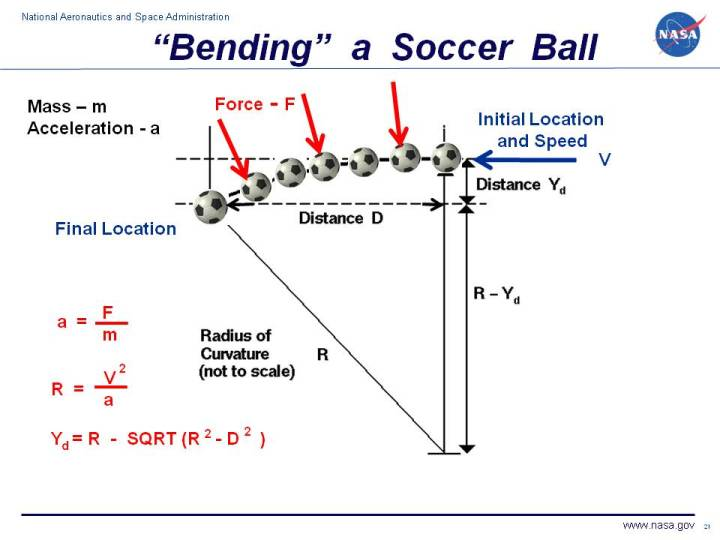+ Text Only Site
+ Non-Flash Version
+ Contact GlennOne of the most exciting plays in the game of soccer is a free kick. Players are often able to curve the flight of the ball into the net by imparting a spin to the ball. Soccer players call this effect "bending" and it is caused by aerodynamic forces on the ball. All that is necessary to create lift is to turn a flow of air. The airfoil of a wing turns a flow, but so does a spinning ball. The details of how the force is generated are fairly complex, but the magnitude of the force F depends on the radius of the ball b, the spin of the ball s, the velocity V of the kick, the density r of the air, and an experimentally determined lift coefficient Cl. F = Cl * 4 /3 * (4 * pi^2 * r * s * V * b^3) where pi is the number 3.14159 .. the ratio of the circumference to the diameter of a circle. As the force acts on the ball, it is deflected along its flight path. If we neglect the viscous forces on the ball, which slow it down and change the magnitude and direction of the force, we have a constant force always acting perpendicular (at a right angle) to the flow direction. The resulting flight path is a circular arc. On the figure, we see the trajectory of the soccer ball as it moves from right to left. The radius of curvature R of the flight path depends on the velocity V of the kick and the acceleration a produced by the side force. R = V^2 / a We can determine this acceleration from Newton's second law of motion using the force for a spinning ball and the mass m of the ball. a = F / m Since the radius of curvature depends on the force, all the factors that affect the force will also affect the trajectory. Collecting all the information into one equation: R = (3 * m * V) / (16 * Cl * r * s * b^3 * pi^2) We can use this equation to make some predictions about the trajectory of a spinning ball. Higher spin s produces a smaller radius of curvature R and a sharper curve. Higher velocity V produces a larger radius of curvarture and a straighter curve. A ball with a smaller mass, like a ping-pong ball, has a lower radius of curvature and curves more. At higher altitudes, the density r is lower producing a larger radius of curvature and a straighter path. The altitude effect helps to explain some of the complaints at the recent World Cup, 2010. The games were played at 10 different stadiums, some at sea level and some high in the mountains. It is much harder to bend a kick at high altitude. Knowing the radius of curvature and the distance of the kick D we can also calculate the distance that the ball is deflected (Yd) along the flight path. There is a right triangle formed by the radius of curvature R, the distance D at the top, and the radius of curvature minus the deflection distance R - Yd on the right. We can then use the Pythagorean Theorem to relate the sides of this triangle: R^2 = D^2 + (R - Yd)^2 Now let's do a little algebra: R^2 - D^2 = (R -Yd)^2 sqrt(R^2 - D^2) = R -Yd Yd = R - sqrt(R^2 - D^2) You can investigate the effect of aerodynamics on kicking a soccer ball by using the SoccerNASA Java Applet. Have fun ! You can use the browser "Back" button to return to this page. If you want your own copy of SoccerNASA to play with, you can download it for free. Activities: Guided Tours Navigation ..NASA Soccer Home Page Beginner's Guide Home Page+ Inspector General Hotline + Equal Employment Opportunity Data Posted Pursuant to the No Fear Act + Budgets, Strategic Plans and Accountability Reports + Freedom of Information Act + The President's Management Agenda + NASA Privacy Statement, Disclaimer, and Accessibility CertificationEditor: Nancy Hall NASA Official: Nancy Hall Last Updated: May 05 2015 + Contact Glenn